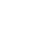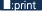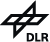# N2O5

There are 5 ascii files containing measured absorption cross sections without wavenumbers. In order to get the wavenumber axis, use:

WAVSTART=540.018915;
´ WAVEND=1380.010840;
WAVSPAC=(wavend-wavstart)/17424;

These wavenumbers correspond to the uncalibrated spectrum. The calibration factor is 1.0000021512. All files have the same number of data points. The absorption cross sections were calculated according to:
-ln(Transmittance)*Temperature[K]/Pressure[mbar]

To convert to cm2/molecule multiply by 5.5448e-21. This contains the conversion from pressure to particle density and the absorption path in the experiment.

The file MESSPAR.DAT contains information linked to the files:

 Air pressure (mbar) Temperature(K) Spectral noise level File name 00.00 205.44 2.91 n2o5205.y 00.00 215.15 0.201 n2o5215.y 00.00 224.97 0.240 n2o5225.y 00.00 254.09 0.302 n2o5255.y 00.00 293.50 0.309 n2o5296.y

We have fitted polynomial coefficients in T (order 2).

The Borland Pascal program abspolyfit.ppp was used to obtain the polynomial coefficients.

Background for calculation of absorption cross sections from polynomial coefficients:

Experimental absorption cross sections for various air pressures and temperatures were fitted by a polynomial in temperature (order 2). The polynomial coefficients are in the file N2O5_POLKOEFF.DAT

The range is ca. 540 - 1380 cm-1.

The input files contain the wavenumber in double precision (8 byte) and 3 polynomial coefficients in single precision (4 byte).
( nu,p(P0,T0),p(P0,T1),p(P0,T2) )

The data are currently binary for LINUX on IBM compatible PC (IEEE format). If you have problems with this format, please contact manfred.birk@dlr.de or georg.wagner@dlr.de . Note: The bytes by may be differently ordered for other than IBM compatible PCs.

The wavenumber grid is determined by the highest resolution experimental spectrum.

Supplied is also a Borland Pascal program calculating desired absorption cross sections for a given air pressure and temperature and spectral range: POL2ABS_N2O5.PP

Furthermore, an ASCII input file named MODEL.INP is given.

689.999393 //start wavenumber
1330.000272 //end wavenumber
13.86 //air pressure in mbar
204.42 //temperature in K
204_012.model //output filename

This file is read by the Pascal program and gives the parameters for the desired absorption cross sections which are outputted in ASCII with calibrated wavenumber and absorption cross section in cm^2/molecule in each line of the output file. Note that the frequency calibration is also carried out in this program.

Receipe for your own software:
1. Select wavenumber range, air pressure (in mbar) and temperature (in K) and calculate ln(pressure +5) (no pressure dependence in case of N2O5)
2. Read in frequency and the polynomial coefficients in the correct order (check "polkeofftype" in our code).
3. Calculate absorption cross sections according to our procedure "model".
4. Do frequency calibration. Calibration factor is given in our code and named "calfact".
5. Enjoy!

Data:
MESSPAR.DAT
MODEL.INP
N2O5_POLKOEFF.DAT
POL2ABS_N2O5.PP
the files listed in the MESSPAR.DAT table above